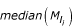# Table 3 Extant scale normalization techniques used in this study. For a given microarray, if M l is a location-normalized log ratio, then M s is the scale-normalized log ratio, where M s = M l / s, and s is median absolute deviation from the median (MAD), a robust estimate of the scale of the data distribution. The remaining abbreviations are as follows, median(M l ): median value of M l values of spots on all microarrays in a data set;: median value of M l values of spots in PT group i on a microarray.

Name * Description Bioconductor R package/function (parameters)
W SCALE Within-microarray scale normalizationM s = M l / s i
marrayNorm/maNormScale (norm="printTipMAD", subset = T, geo = T, Mscale = T)
B SCALE Between-microarray scale normalization
s = median(M l - median(M l ))
M s = M l / s
marrayNorm/maNormScale (norm="globalMAD", subset = T), geo = T, Mscale = T)
WB SCALE Step 1: Within-microarray scale normalizationmarrayNorm/maNormScale (norm="printTipMAD", subset = T, geo = T, Mscale = T)
Step 2: Between-microarray scale normalization
s = median(M l - median(M l ))marrayNorm/maNormScale (norm="globalMAD", subset = T, geo = T, Mscale = T)
1. * We adopted the terminology given in this table to avoid confusion within this work. Elsewhere, the methods are known as: W SCALE, within-print-tip-group scale normalization ; and B SCALE, between slide scale normalization [4, 15].